## Using the Properties of Trapezoids to Solve Problems

### Learning Outcomes

• Use properties of trapezoids

A trapezoid is a four-sided figure, a quadrilateral, with two sides that are parallel and two sides that are not. The parallel sides are called the bases. We call the length of the smaller base $b$, and the length of the bigger base $B$. The height, $h$, of a trapezoid is the distance between the two bases as shown in the image below.

A trapezoid has a larger base, $B$, and a smaller base, $b$. The height $h$ is the distance between the bases.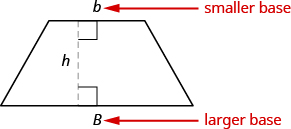The formula for the area of a trapezoid is:

${\text{Area}}_{\text{trapezoid}}=\Large\frac{1}{2}\normalsize h\left(b+B\right)$

Splitting the trapezoid into two triangles may help us understand the formula. The area of the trapezoid is the sum of the areas of the two triangles. See the image below.

Splitting a trapezoid into two triangles may help you understand the formula for its area.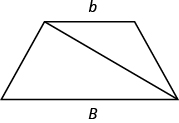The height of the trapezoid is also the height of each of the two triangles. See the image below.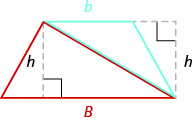The formula for the area of a trapezoid is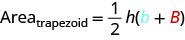If we distribute, we get,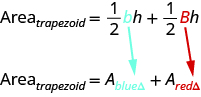### Properties of Trapezoids

• A trapezoid has four sides.
• Two of its sides are parallel and two sides are not.
• The area, $A$, of a trapezoid is $\text{A}=\Large\frac{1}{2}\normalsize h\left(b+B\right)$ .

### example

Find the area of a trapezoid whose height is $6$ inches and whose bases are $14$ and $11$ inches.

Solution

 Step 1. Read the problem. Draw the figure and label it with the given information.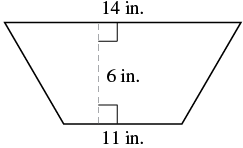Step 2. Identify what you are looking for. the area of the trapezoid Step 3. Name. Choose a variable to represent it. Let $A=\text{the area}$ Step 4.Translate. Write the appropriate formula. Substitute.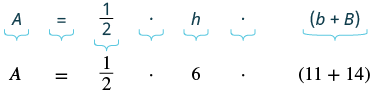Step 5. Solve the equation. $A={\Large\frac{1}{2}}\normalsize\cdot 6(25)$ $A=3(25)$ $A=75$ square inches Step 6. Check: Is this answer reasonable? $\checkmark$  see reasoning below

If we draw a rectangle around the trapezoid that has the same big base $B$ and a height $h$, its area should be greater than that of the trapezoid.
If we draw a rectangle inside the trapezoid that has the same little base $b$ and a height $h$, its area should be smaller than that of the trapezoid.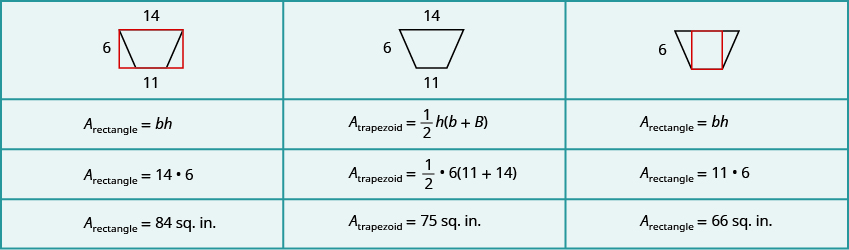The area of the larger rectangle is $84$ square inches and the area of the smaller rectangle is $66$ square inches. So it makes sense that the area of the trapezoid is between $84$ and $66$ square inches

Step 7. Answer the question. The area of the trapezoid is $75$ square inches.

### try it

In the next video we show another example of how to use the formula to find the area of a trapezoid given the lengths of it’s height and bases.

### example

Find the area of a trapezoid whose height is $5$ feet and whose bases are $10.3$ and $13.7$ feet.

### example

Vinny has a garden that is shaped like a trapezoid. The trapezoid has a height of $3.4$ yards and the bases are $8.2$ and $5.6$ yards. How many square yards will be available to plant?

## Contribute!

Did you have an idea for improving this content? We’d love your input.